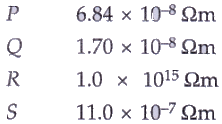# Solutions of Electricity (Page No- 67) - Physics By Lakhmir Singh, Class 10 Class 10 Notes | EduRev

## Class 10 : Solutions of Electricity (Page No- 67) - Physics By Lakhmir Singh, Class 10 Class 10 Notes | EduRev

The document Solutions of Electricity (Page No- 67) - Physics By Lakhmir Singh, Class 10 Class 10 Notes | EduRev is a part of the Class 10 Course Class 10 Physics Solutions By Lakhmir Singh & Manjit Kaur.
All you need of Class 10 at this link: Class 10

Lakhmir Singh Physics Class 10 Solutions Page No:67

Question 31:
The electrical resistivities of four materials P, Q, R and S are given below :Which material will you use for making : (a) heating element of electric iron (b) connecting wires of electric iron (c) covering of connecting wires ? Give reason for your choice in each case.
Solution :
(a) S; because it has high resistivity of 11/10000000 ohm-m (it is actually nichrome).
(b) Q; because it has very low resistivity of 1.7/100000000 ohm-m (it is actually copper).
(c) R; because it has very very high resistivity of 1.0X100000000000000 ohm-m (it is actually rubber).

Question 32:
(a) How does the wire in the filament of a light bulb behave differently to the other wires in the circuit when the current flows ?
(b) What property of the filament wire accounts for this difference ?

Solution :
(a) The filament wire becomes white hot where as other wires in the circuit do not get heated much.
(b) High resistance of filament wire accounts for this difference.

Question 33:
Two exactly similar heating resistances are connected (i) in series, and (ii) in parallel, in two different circuits, one by one. If the same current is passed through both the combinations, is more heat obtained per minute when they are connected in series or when they are connected in parallel ? Give reason for your answer.

Solution :
In series, because total resistance in series connection is more than that in parallel connection.

Question 34:
An electric iron is connected to the mains power supply of 220 V. When the electric iron is adjusted at ‘minimum heating’ it consumes a power of 360 W but at ‘maximum heating’ it takes a power of 840 W. Calculate the current and resistance in each case.

Solution :

Given: V = 220V. Pmin=360W. Pmax = 840W
For minimum heating case:
We know that
Pmin = VI
360 = 220X1
I = 1.63amp
R = V/I
R = 22011.63
R =134.96ohms

For maximum heating case:
We know that
Pmax = VI
840 = 220X1
I = 3.81amp
R = V/I
R = 220/3.81
R = 57.74ohms

Question 35:
Which electric heating devices in your home do you think have resistors which control the flow of electricity ?

Solution :
Electric iron , electric oven, water heater, room heater.

Offer running on EduRev: Apply code STAYHOME200 to get INR 200 off on our premium plan EduRev Infinity!

94 docs

,

,

,

,

,

,

,

,

,

,

,

,

,

,

,

,

,

,

,

,

,

,

,

,

;